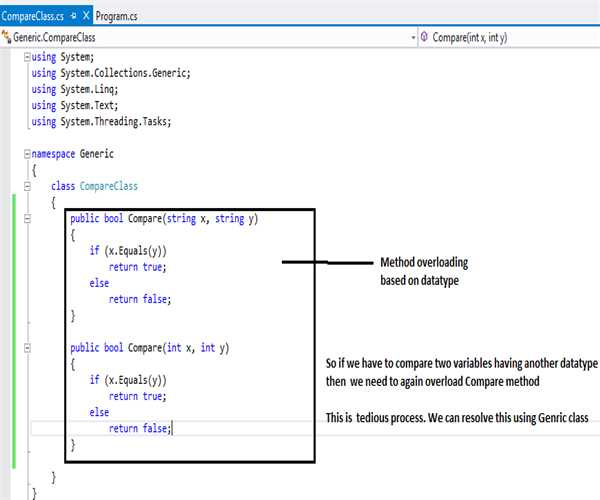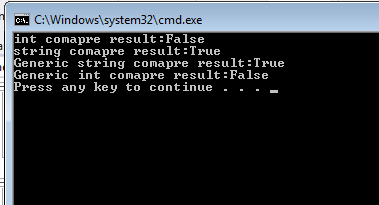##### Introduction

I would like to share basic of generics. Here first we will learn Problem statement

and then will resolve the problem using Generics.

##### Problem statement:

First create a class as per following code.

`class CompareClass    {        public bool Compare(string x, string y)        {            if (x.Equals(y))                return true;            else                return false;        }         public bool Compare(int x, int y)        {            if (x.Equals(y))                return true;            else                return false;        }     }`

##### Understanding the code
1. We created class CompareClass
2. Here we created two compare methods, one for string data type and second
3. for int data type.
4. So class contains overloaded compare functions.
##### Problem
• So if we need to compare other datatype like decimal, double, objects then above code would not work and we have to create another method to compare for proposed data type.
• We can solve this problem with generic.Solution:

Create a class as per below code

`class CompareGenericClass<T>    {        public bool Compare(T x, T y)        {            if (x.Equals(y))                return true;            else                return false;        }     }`
##### Understanding the code
• We created class CompareGenericClass with input parameter as T, so class is
• CompareGenericClass<T>

Here T would act as datatype.

• If we want to compare strings then following style would be used to create

object of class

`CompareGenericClass<string> Ocompare = new CompareGenericClass<string>();       bool stringResult=Ocompare.Compare("DEVESH", "DEVESH");`1. As we passed T as string then Compare method will accept only string type of

parameter.

1. Similar we did for Integer as well.
`CompareGenericClass<int> oIntcompare = new CompareGenericClass<int>();         bool integerresult=oIntcompare.Compare(5, 6);`1. Here with this class we do not need to overload Compare method because

this compare method accepts only parameter which has been passed during

creation of class objects.

1. Using this class we can avoid such problems which have been discussed

above.

Output of code.Complete code

`    class CompareClass    {        public bool Compare(string x, string y)        {            if (x.Equals(y))                return true;            else                return false;        }         public bool Compare(int x, int y)        {            if (x.Equals(y))                return true;            else                return false;        }     }      class CompareGenericClass<T>    {        public bool Compare(T x, T y)        {            if (x.Equals(y))                return true;            else                return false;        }     }class Program    {        static void Main(string[] args)        {            CompareClass obj = new CompareClass();            bool intresult=obj.Compare(5, 7);            Console.WriteLine("int comapre result:" + intresult);             bool stringresult = obj.Compare("DEVESH", "DEVESH");            Console.WriteLine("string comapre result:" +stringresult);                                              CompareGenericClass<string> Ocompare = new CompareGenericClass<string>();            bool stringResult=Ocompare.Compare("DEVESH", "DEVESH");             Console.WriteLine("Generic string comapre result:" + stringResult);             CompareGenericClass<int> oIntcompare = new CompareGenericClass<int>();             bool integerresult=oIntcompare.Compare(5, 6);             Console.WriteLine("Generic int comapre result:" + integerresult);         }`

Conclusion

We have learned how to use Generic class and why we have to use.

Modified On Sep-07-2019 12:14:41 AM
1.Thank You.